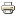Article
Author/s:
Takashi Hayashi, Michele Lombardi
Journal of Mathematical Economics
Issue number:
Volume 83, August 2019
Publisher:
Elsevier
Year:
2019
Journal pages:
110-126
In many situations, agents are involved in an allocation problem that is followed by another allocation problem whose optimal solution depends on how the former problem has been solved. In this paper, we take this dynamic structure of allocation problems as an institutional constraint. By assuming a finite number of allocation problems, one for each period/stage, and by assuming that all agents in society are involved in each allocation problem, a dynamic mechanism is a period-by-period process. This process generates at any period- history a period- mechanism with observable actions and simultaneous moves. We also assume that the objectives that a planner wants to achieve are summarized in a social choice function (SCF), which maps each state (of the world) into a period-by-period outcome process. In each period , this process selects for each state a period- socially optimal outcome conditional on the complete outcome history realized up to period . Heuristically, the SCF is one-step-ahead implementable if there exists a dynamic mechanism such that for each state and each realized period- history, each of its subgame perfect Nash equilibria generates a period-by-period outcome process that coincides with the period-by-period outcome process that the SCF generates at that state from period onwards. We identify a necessary condition for SCFs to be one-step-ahead implemented, one-step-ahead Maskin monotonicity, and show that it is also sufficient under a variant of the condition of no veto-power when there are three or more agents. Finally, we provide an account of welfare implications of one-step-ahead implementability in the contexts of trading decisions and voting problems.
Developed by Paolo Gittoi